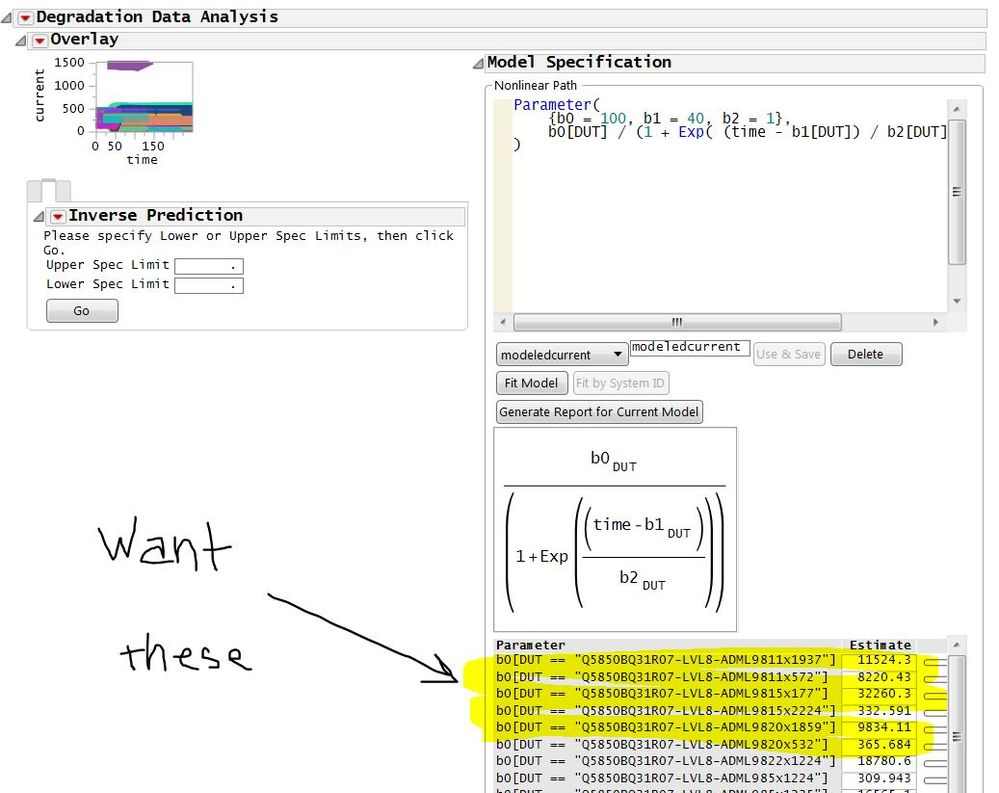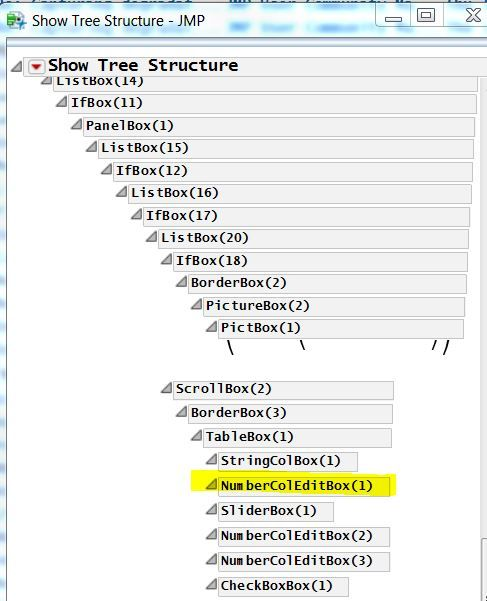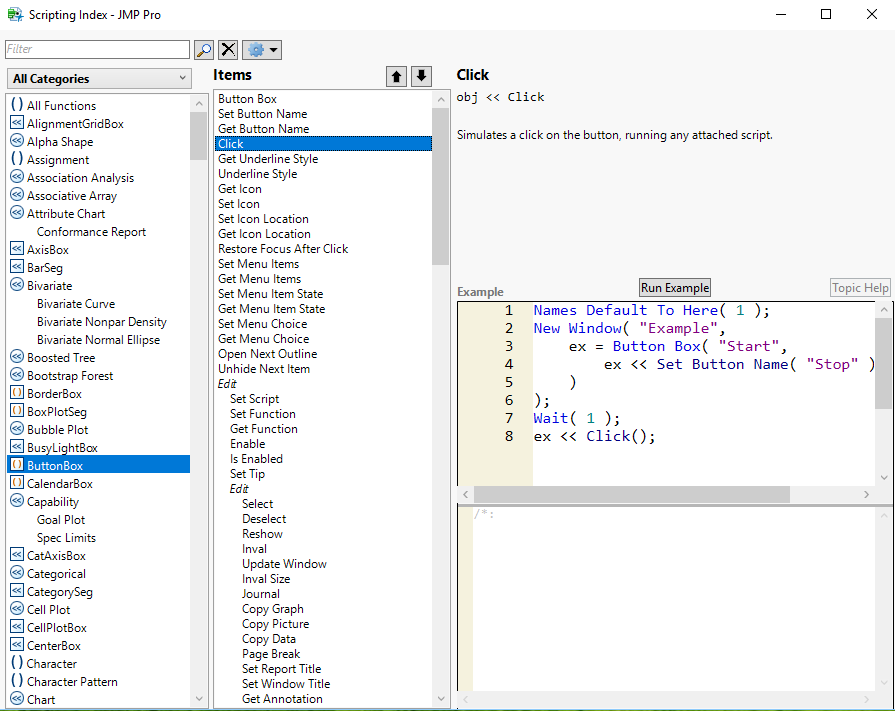Choose Language Hide Translation Bar
Highlighted

## Capturing degradation fit parameters to table?

How do I capture reliability degradation fit parameters to table?

The four bolded lines are the crux of what I am trying to do.

Fitting the degradation and then simply capturing the fit parameters in a column.

Script below:

Y( :current ),
Time( :time ),
Label( :DUT ),
Connect Data Markers( 1 ),
Show Fitted Lines( 0 ),
Show Spec Limits( 1 ),
Show Median Curves( 0 ),
Show Legend( 0 ),
No Tab List( 0 ),
Set Upper Spec Limit( . ),
Set Lower Spec Limit( . ),
Set Censoring Time( . ),
Show Residual Plot( 1 ),
Show Inverse Prediction Plot( 1 ),
Inverse Prediction Interval( No Interval ),
Inverse Prediction Alpha( 0.05 ),
Path Specifications(
Simple Linear(
{{"Linear", Function( {x}, x ), Function( {x}, x )}}
),
{{"Linear", Function( {x}, x ), Function( {x}, x )}}
),
Slope( Different ),
Intercept( Different ),
Select X Scale( "Linear" ),
Select Y Scale( "Linear" )
),
Nonlinear Path(
Formula Name( "modeledcurrent" ),
Formula(
Parameter(
{b0 = 100, b1 = 40, b2 = 1},
b0[DUT] / (1 + Exp( (time - b1[DUT]) / b2[DUT] ))
)
),
Fitting Method( QuasiNewton BFGS ),
Fixed(
[0, 0, 0, 0, 0, 0, 0, 0, 0, 0, 0, 0, 0, 0, 0, 0, 0, 0, 0, 0, 0,
0, 0, 0, 0, 0, 0, 0, 0, 0, 0, 0, 0, 0, 0, 0, 0, 0, 0, 0, 0, 0, 0,
0, 0, 0, 0, 0, 0, 0, 0, 0, 0, 0, 0, 0, 0, 0, 0, 0, 0, 0, 0, 0, 0,
0]
)
),
Select Formula( "modeledcurrent" )
)
),
Nonlinear Path( 1 ),
Mean Path( 1 ),
SendToReport(
Dispatch(
{"Overlay", "current Residuals by time"},
"7",
ScaleBox,
{Min( -350 ), Max( 325 ), Inc( 50 ), Minor Ticks( 1 )}
),
Dispatch(
{"Overlay", "current Residuals by time"},
"13",
ScaleBox,
{Min( -350 ), Max( 325 ), Inc( 50 ), Minor Ticks( 1 )}
),
Dispatch(
{"Overlay", "Model Specification"},
"Empty",
TextEditBox,
{Fixed Size( 1, 100, 14 ), Set Text( "modeledcurrent" )}
)
)
);

Caption( "Picking off parameters from MPD curves" );
Wait( 0 );
prgStatus = New Window( "Progress",
Show Toolbars( 0 ),
V List Box(
align( center ),
dlg_gb = Graph Box(
Title( "200hr ttfs from MPD curves" ),
FrameSize( 200, 30 ),
X Scale( 0, 100 ),
Y Scale( 0, 1 ),
xaxis( Inside Ticks( 1 ) ),
yaxis( Show Major Ticks( 0 ), Show Minor Ticks( 0 ), Show Labels( 0 ) ),
xname( "% Complete" ),
yname( "" )
),
Button Box( "Cancel", prgStatus << Close Window )
)
);

dt = Current Data Table();
p# = N Row( dt );
dt << New Column( "bee0", Numeric, Continuous, Format( "Fixed Dec", 8, 1 ) );
dt << New Column( "bee1", Numeric, Continuous, Format( "Fixed Dec", 8, 1 ) );
dt << New Column( "bee2", Numeric, Continuous, Format( "Fixed Dec", 8, 1 ) );

For( i = 1, i <= p#, i++,
prgrs = (i / p#) * 100;
Try(
prgStatus[FrameBox( 1 )] <<
Add Graphics Script( {Fill Color( "green" ), Rect( 0, 1, prgrs, 0, 1 )} ),
i = p# + 1
);
Wait( 0 );
Try(:bee0[i]=b0[:DUT[i]]);
Try(:bee1[i]=b1[:DUT[i]]);
Try(:bee2[i]=b2[DUT[i]])

);

How do I access the fit parameters?

10 REPLIES 10
Highlighted

## Re: Capturing degradation fit parameters to table?

@galactus3000,

https://community.jmp.com/t5/JSL-Cookbook/Extract-Statistics-from-an-Analysis-into-a-Report/ta-p/415...

Best
Uday
Highlighted

## Re: Capturing degradation fit parameters to table?

thanks ... closer but not there yet .... how do I now extract the values I want?

script below ... I've created analysis and report level variables dgr and reportdgr

but now how do I get the parameters from this variable reportdgr? (see pic below script)

``````dt=currentdatatable();

Y( :current ),
Time( :time ),
Label( :DUT ),
Connect Data Markers( 1 ),
Show Fitted Lines( 0 ),
Show Spec Limits( 1 ),
Show Median Curves( 0 ),
Show Legend( 0 ),
No Tab List( 0 ),
Set Upper Spec Limit( . ),
Set Lower Spec Limit( . ),
Set Censoring Time( . ),
Show Residual Plot( 1 ),
Show Inverse Prediction Plot( 1 ),
Inverse Prediction Interval( No Interval ),
Inverse Prediction Alpha( 0.05 ),
Path Specifications(
Simple Linear(
{{"Linear", Function( {x}, x ), Function( {x}, x )}}
),
{{"Linear", Function( {x}, x ), Function( {x}, x )}}
),
Slope( Different ),
Intercept( Different ),
Select X Scale( "Linear" ),
Select Y Scale( "Linear" )
),
Nonlinear Path(
Formula Name( "modeledcurrent" ),
Formula(
Parameter(
{b0 = 100, b1 = 40, b2 = 1},
b0[DUT] / (1 + Exp( (time - b1[DUT]) / b2[DUT] ))
)
),
Fitting Method( QuasiNewton BFGS ),
Fixed(
[0, 0, 0, 0, 0, 0, 0, 0, 0, 0, 0, 0, 0, 0, 0, 0, 0, 0, 0, 0, 0,
0, 0, 0, 0, 0, 0, 0, 0, 0, 0, 0, 0, 0, 0, 0, 0, 0, 0, 0, 0, 0, 0,
0, 0, 0, 0, 0, 0, 0, 0, 0, 0, 0, 0, 0, 0, 0, 0, 0, 0, 0, 0, 0, 0,
0]
)
),
Select Formula( "modeledcurrent" )
)
),
Nonlinear Path( 1 ),
Mean Path( 1 )
//	,SendToReport(
//		Dispatch(
//			{"Overlay", "current Residuals by time"},
//			"7",
//			ScaleBox,
//			{Min( -350 ), Max( 325 ), Inc( 50 ), Minor Ticks( 1 )}
//		),
//		Dispatch(
//			{"Overlay", "current Residuals by time"},
//			"13",
//			ScaleBox,
//			{Min( -350 ), Max( 325 ), Inc( 50 ), Minor Ticks( 1 )}
//		),
//		Dispatch(
//			{"Overlay", "Model Specification"},
//			"Empty",
//			TextEditBox,
//			{Fixed Size( 1, 100, 14 ), Set Text( "modeledcurrent" )}
//		)
//	)
);
reportdgr=dgr<<Report;
reportdgr[Outline Box("Overlay")][Outline Box("Model Specification")]<<close(0);``````Highlighted

## Re: Capturing degradation fit parameters to table?

Use Show Tree Structure() . This should give you the reference name of the Parameter block in your window.

You can then extract values by referring to that box

https://community.jmp.com/t5/Discussions/How-to-extract-Parameter-Estimates-of-Reciprocals-i-e-make-...

Best
Uday
Highlighted

## Re: Capturing degradation fit parameters to table?

ah ... so I am primarily interested in NumberColEditBox(1) highlighted belowHighlighted

## Re: Capturing degradation fit parameters to table?

In order to fit the model, I need to "press" ButtonBox(5) which is labeled Fit Model

how do I do this?

I tried

``dgr<<Fit Model;``

but this didn't work?

Highlighted

## Re: Capturing degradation fit parameters to table?

still looking for answers on this

Highlighted

## Re: Capturing degradation fit parameters to table?

Try 'Help > Scripting Index' then search for "Button Box". You should see that you can send it a 'Click' message which has the same effect as pressing the button manually.

Highlighted

## Re: Capturing degradation fit parameters to table?

Ian,

Thanks for the response.  Upon refering to scripting index, the <<click command for ButtonBox seems elusive.  Not seeing it.

Charlie

Highlighted

## Re: Capturing degradation fit parameters to table?

Here is a screen capture from the Scripting Index that details the "Click" for the Button Box()Jim
Article Labels

There are no labels assigned to this post.